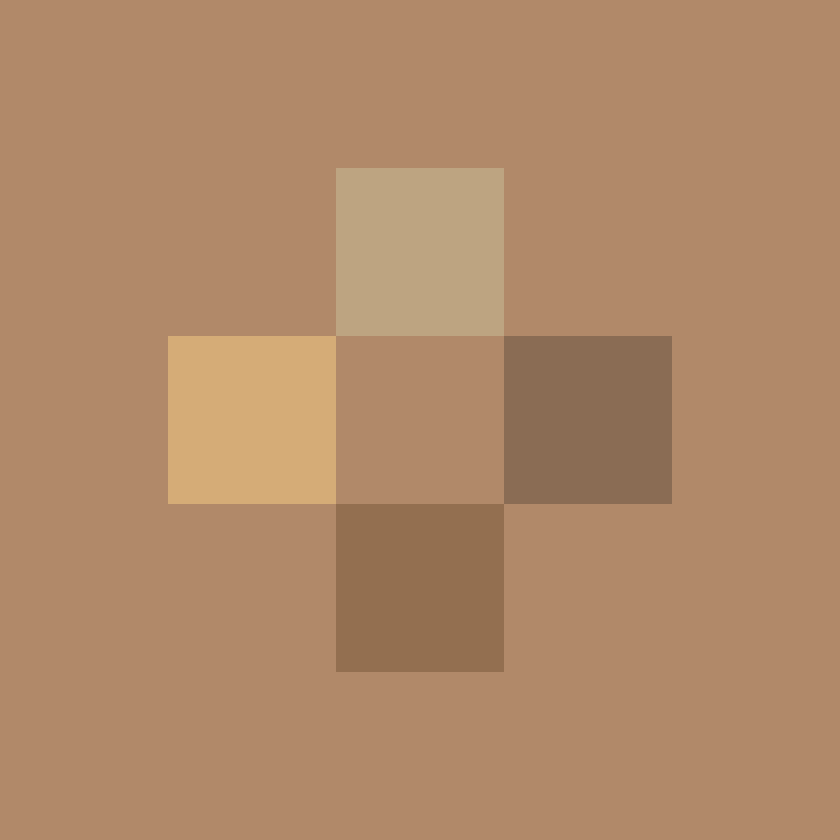# Generating 3D Interactive Models¶

In the same way that single lines of code in Python based notebooks can be used to generate and render rich interactive media as HTML+Javascript code cell outputs, so too can R notebooks by building on the htmlwidgets R package.

Note

Interactive views can also be generated as chunk outputs in RStudio and rendered into output HTML documents using packages such as knitr and bookdown; the latter provides a publishing workflow akin to that of Jupyter Book.

options(rgl.useNULL = TRUE,
rgl.printRglwidget = TRUE)


## Interactive 3D Models with rgl¶

The rgl package ..

library(rgl)

rgl::clear3d()

simple_matrix = matrix(c(0, 0, 0, 0, 0,
0, 0, 0, 0, 0,
0, 0, 5, 0, 0,
0, 0, 0, 0, 0,
0, 0, 0, 0, 0), 5)

simple_map = simple_matrix %>%
sphere_shade(texture = "desert", progbar = FALSE)

simple_map %>%
plot_map()simple_map %>%
plot_3d(simple_matrix, linewidth=0)

rgl::rglwidget()